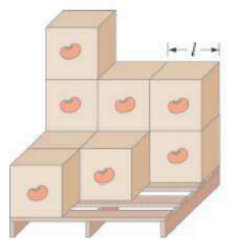×
Get Full Access to Physics: Principles With Applications - 6 Edition - Chapter 7 - Problem 51p
Get Full Access to Physics: Principles With Applications - 6 Edition - Chapter 7 - Problem 51p

×

# (II) A (lightweight) pallet has a load of ten identicalISBN: 9780130606204 3

## Solution for problem 51P Chapter 7

Physics: Principles with Applications | 6th Edition

• Textbook Solutions
• 2901 Step-by-step solutions solved by professors and subject experts
• Get 24/7 help from StudySoup virtual teaching assistantsPhysics: Principles with Applications | 6th Edition

4 5 1 425 Reviews
24
2
Problem 51P

(II) A (lightweight) pallet has a load of identical cases of tomato paste (see Fig.), each of which is a cube of length. Find the center of gravity in the horizontal plane, so that the crane operator can pick up the load without tipping it.FIGURE 7-40 Problem 51

Step-by-Step Solution:

We have to determine the location of the center of gravity (center of mass) of the systems in the horizontal.

Step 1 of 3

Concept:

Center of mass(CM) of a body is an imaginary point at which the whole mass of the body is supposed to be concentrated. We consider that the net force acts on the center of mass of the body, instead of considering that it acts on each and every point on the body for simpler calculation and this is one of the importance of the application of CM.

Center of the gravity of a body is an imaginary point at which the whole weight of the body is acting vertically downward. For the body with uniform distribution and placed in a uniform gravitational field, the center of mass of the body coincides with the center of gravity.

Step 2 of 4

Step 3 of 4

## Discover and learn what students are asking

Unlock Textbook Solution